### Home > CALC > Chapter 5 > Lesson 5.2.1 > Problem5-57

5-57.
1.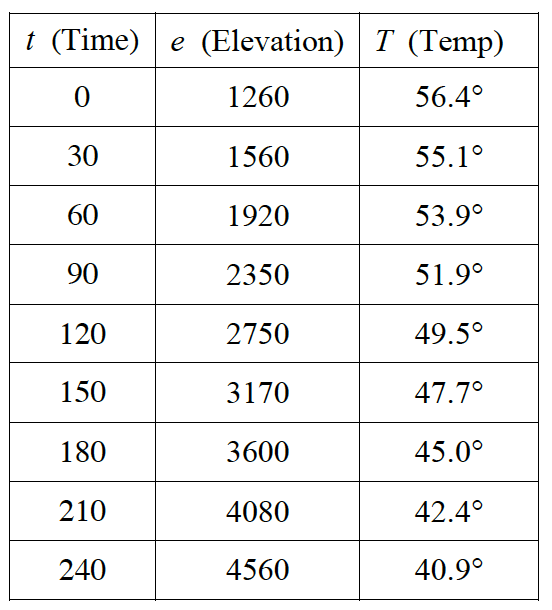After a weather balloon is launched the following data is sent back to the meteorological center. The column marked time, t, is given in seconds after launch, elevation, e, in feet above sea level, and temperature, T, in degrees Fahrenheit. Homework Help ✎

1. Approximately how fast (in ft/sec) is the balloon rising at 120 seconds?

2. Approximately how fast is the temperature changing (in °F/ft) at 2750 feet?

3. Approximately how fast is the temperature changing (in °F/sec) at 120 seconds?

4. Why would you expect the product of the answers to (a) and (b) to equal answer (c)?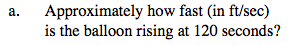Since we cannot find the derivative (IROC), calculate the average rate of change.
For e(t):

$\text{You could use Hana's method: }\frac{e(150)-e(120)}{150-120}$

$\text{or Anah's method: }\frac{e(120)-e(90)}{120-90}$

$\text{or Hanah's method: }\frac{e(150)-e(90)}{150-90}$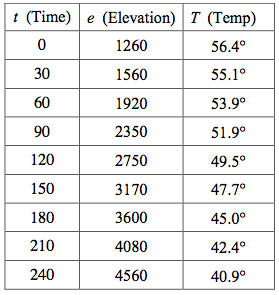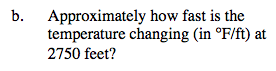Use Hana, Anah, or Hanah's method to approximate the rate of change for T(e) at e = 2750.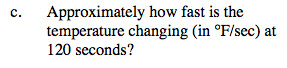Use Hana, Anah, or Hanah's method to approximate the rate of change for T(t) at t = 120.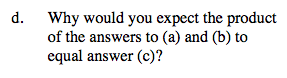$\text{Rewrite: }e'(t)=\frac{de}{dt} \text{ and }T'(e)=\frac{dT}{de}$

$\frac{de}{dt}\cdot \frac{dT}{de}=$